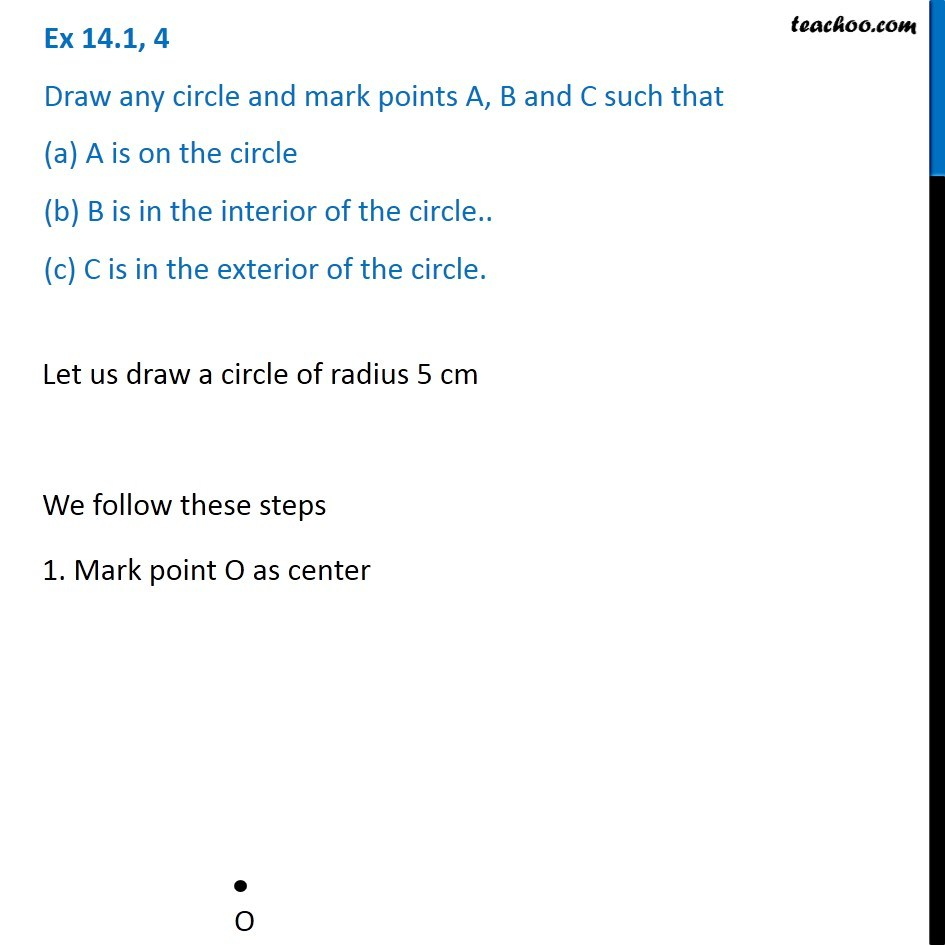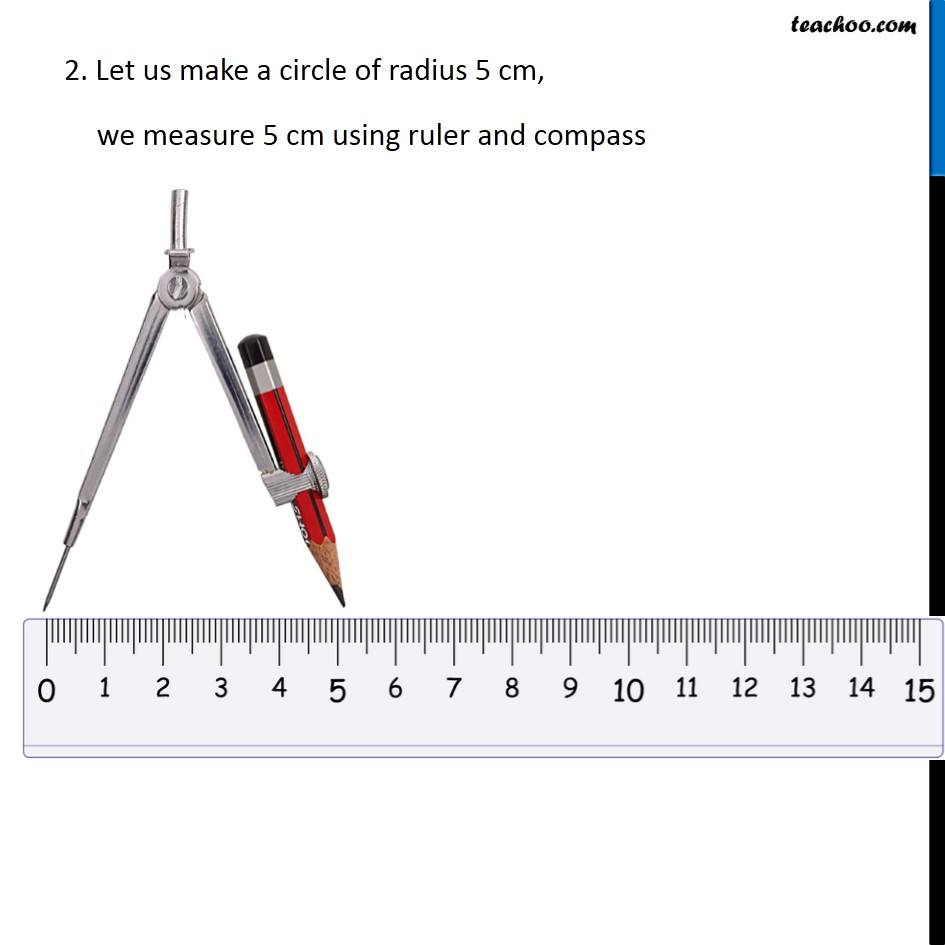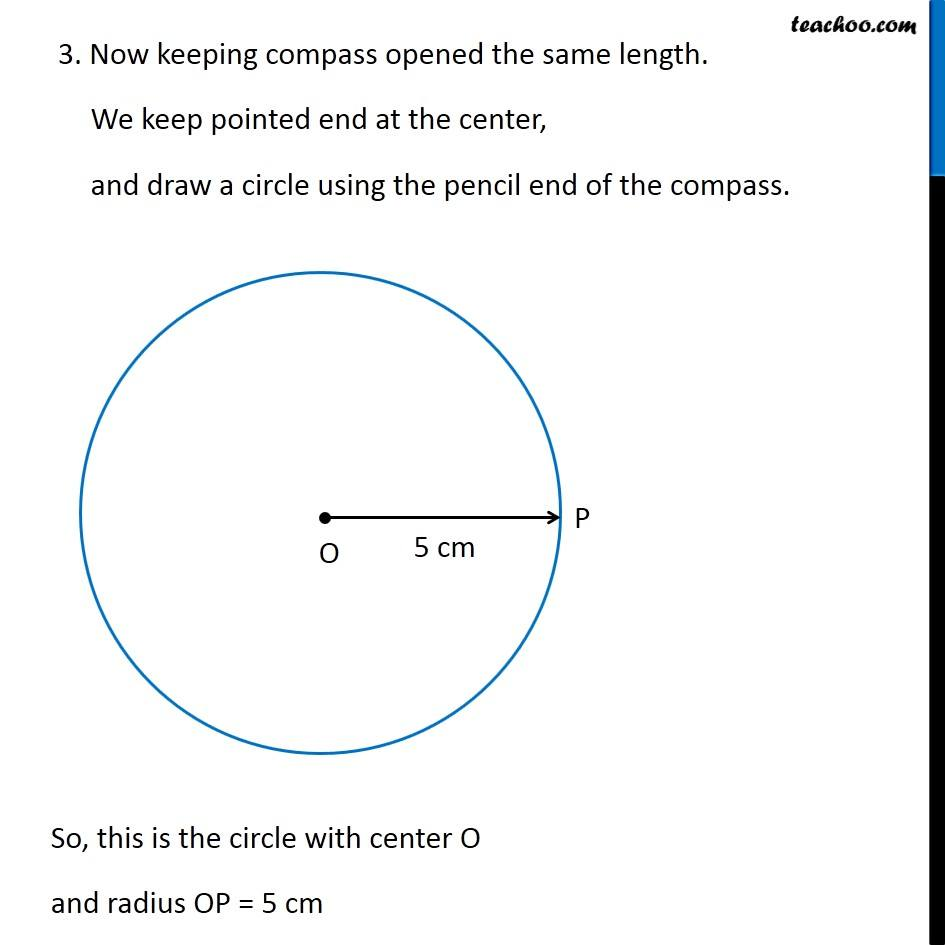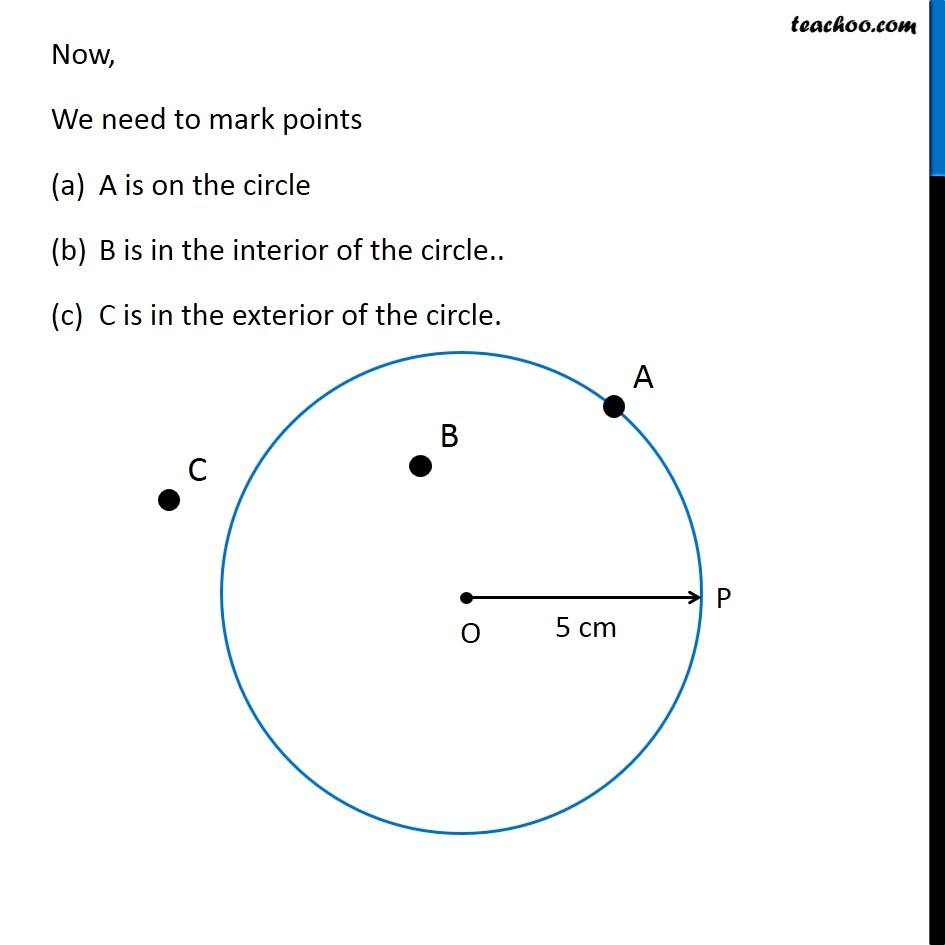Constructing a circle

Practical Geometry
Serial order wiseLearn in your speed, with individual attention - Teachoo Maths 1-on-1 Class

### Transcript

Question 4 Draw any circle and mark points A, B and C such that (a) A is on the circle (b) B is in the interior of the circle.. (c) C is in the exterior of the circle. Let us draw a circle of radius 5 cm We follow these steps 1. Mark point O as center 2. Let us make a circle of radius 5 cm, we measure 5 cm using ruler and compass 3. Now keeping compass opened the same length. We keep pointed end at the center, and draw a circle using the pencil end of the compass. So, this is the circle with center O and radius OP = 5 cm Now, We need to mark points A is on the circle B is in the interior of the circle.. C is in the exterior of the circle.